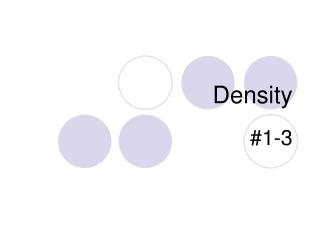DownloadDownload PresentationDensity

# Density

Télécharger la présentation## Density

- - - - - - - - - - - - - - - - - - - - - - - - - - - E N D - - - - - - - - - - - - - - - - - - - - - - - - - - -
##### Presentation Transcript

1. Density #1-3

2. What is density? • Density is a comparison of how much matter there is in a certain amount of space.

3. Which one is more dense? • Demonstration: People in a square • How about this: Which square is more dense?

4. Which one is more dense? • Now which one is more dense?

5. What is density? • Density = mass OR mass ÷ volume. volume • Units for density: g . cm3 • Why are these the units for density? ALWAYS REMEMBER UNITS!

6. Let’s try a density problem together • Frank has a paper clip. It has a mass of 9g and a volume of 3cm3. What is its density? • Frank also has an eraser. It has a mass of 3g, and a volume of 1cm3. What is its density?

7. Work on these problems with your neighbor. • Jack has a rock. The rock has a mass of 6g and a volume of 3cm3. What is the density of the rock? • Jill has a gel pen. The gel pen has a mass of 8g and a volume of 2cm3. What is the density of the rock?

8. Now, try these on your own. • Al’Licia has a watch. It has a mass of 4g and a volume of 2cm3. What is the density of the watch? • Mia has a wallet. It has a mass of 15g and a volume of 5cm3. What is the density of the wallet?

9. Liquid Layers • If you pour together liquids that don’t mix and have different densities, they will form liquid layers. • The liquid with the highest density will be on the bottom. • The liquid with the lowest density will be on the top.

10. Liquid Layers • Check out this picture from your book. Which layer has the highest density? • Which layer has the lowest density? • Imagine that the liquids have the following densities: • 10g/cm3. 3g/cm3. • 6g/cm3. 5g/cm3. • Which number would go with which layer?

11. Liquid Layers – Try with your neighbor • Which liquid has the highest density? • Which liquid has the lowest density? • Which liquid has the middle density?

12. Liquid Layers – Try on your own! • Imagine that the liquids on the right have the following densities: • 15g/cm3 10g/cm3 • 3g/cm3 9g/cm3 • 7g/cm3 12g/cm3 • Match the colors to the correct densities. 3g/cm3 7g/cm3 9g/cm3 10g/cm3 12g/cm3 15g/cm3

13. Review • What is the formula for density? • What happens if you pour together liquids that have different densities? • Will the liquid on the top have the highest or lowest density? • Will the liquid on the bottom have the highest or lowest density?

14. Super Scientist Question of the Day • Jake has a book, a ruler, and a balance. • How can Jake find the density of the book with the tools he has?